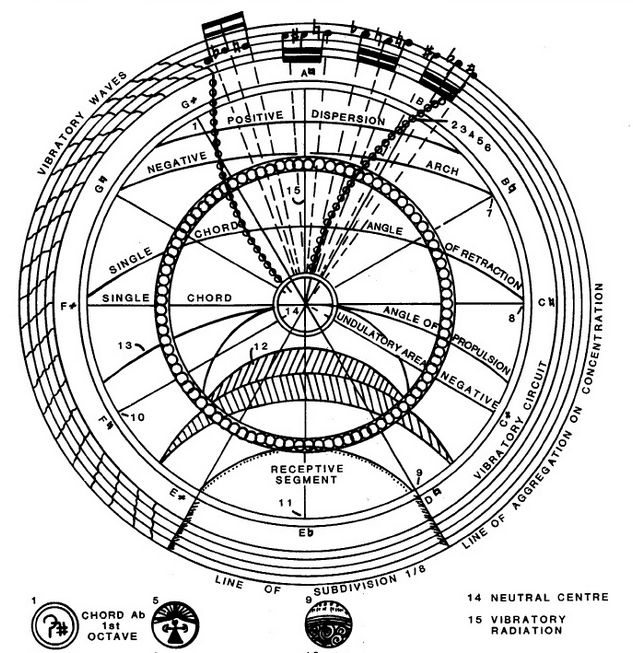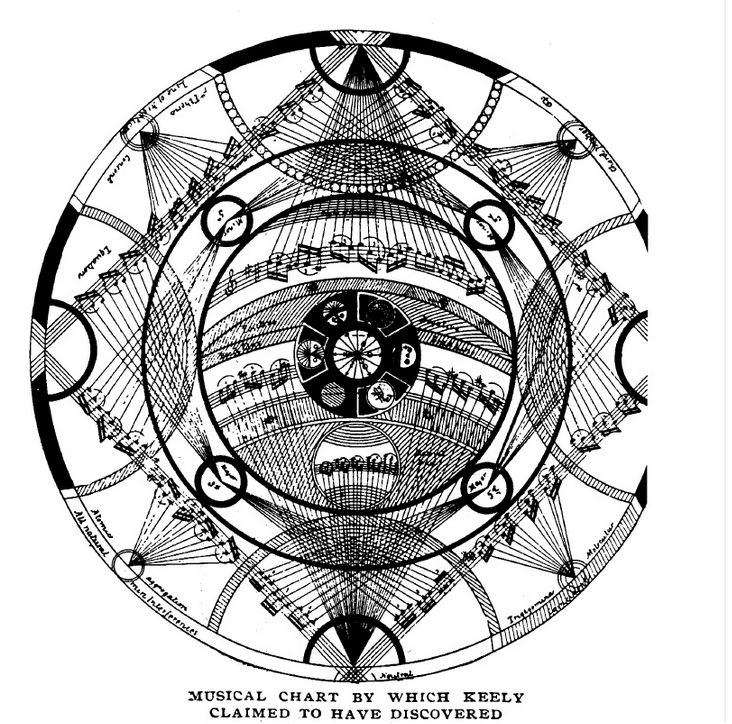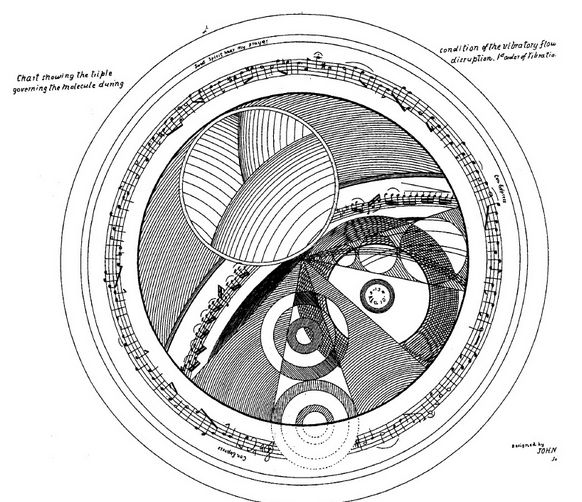rexresearch.com

John KEELY
Atomic / Etheric Triplets

These are mp3 files generated by Audacity freeware ( available here ), based on Keely's drawings of his acoustic model of atoms and molecules.
More will be added as time allows.
The tones are in the A = 432 Hz Equal Tempered Scale :

Note 8ve = Frequency (Hz)

C0 = 16.05
C#0 / Db0 = 17.01
D0 = 18.02
D#0 / Eb0 = 19.09
E0 = 20.23
F0 = 21.43
F#0 / Gb0 = 22.70
G0 = 24.05
G#0 / Ab0 = 25.48
A0 = 27.00
A#0 / Bb0 = 28.61
B0 = 30.31
C1 = 32.11
C#1 / Db1 = 34.02
D1 = 36.04
D#1 / Eb1 = 38.18
E1 = 40.45
F1 = 42.86
F#1 / Gb1 =45.41
G1 = 48.11
G#1 / Ab1 = 50.97
A1 = 54.00
A#1 / Bb1 = 57.21
B1 = 60.61
C2 = 64.22
C#2 / Db2 = 68.04
D2 = 72.08
D#2 / Eb2 = 76.37
E2 = 80.91
F2 = 85.72
F#2 / Gb2 = 90.82
G2 = 96.22
G#2 / Ab2 = 101.94
A2 = 108.00
A#2 / Bb2 = 114.42
B2 = 121.23
C3 = 128.43
C#3 / Db3 = 136.07
D3 = 144.16
D#3 / Eb3 = 152.74
E3 = 161.82
F3 = 171.44
F#3 / Gb3 = 181.63
G3 = 192.43
G#3 / Ab3 = 203.88
A3 = 216.00
A#3 / Bb3 = 228.84
B3 = 242.45
C4 = 256.87
C#4 / Db4 = 272.14
D4 = 288.33
D#4 / Eb4 = 305.47
E4 = 323.63
F4 = 342.88
F#4 / Gb4 = 363.27
G4 = 384.87
G#4 / Ab4 = 407.75
A4 = 432.00
A#4 / Bb4 = 457.69
B4 = 484.90
C5 = 513.74
C#5 / Db5 = 544.29
D5 = 576.65
D#5 / Eb5 = 610.94
E5 = 647.27
F5 = 685.76
F#5 / Gb5 = 726.53
G5 = 769.74
G#5 / Ab5 = 815.51
A5 = 864.00
A#5 / Bb5 = 915.38
B5 = 969.81
C6 = 1027.47
C#6 / Db6 = 1088.57
D6 = 1153.30
D#6 / Eb6 = 1221.88
E6 = 1294.54
F6 = 1371.51
F#6 / Gb6 = 1453.07
G6 = 1539.47
G#6 / Ab6 = 1631.01
A6 = 1728.00
A#6 / Bb6 = 1830.75
B6 = 1939.61
C7 = 2054.95
C#7 / Db7 = 2177.14
D7 = 2306.60
D#7 / Eb7 = 2443.76
E7 = 2589.07
F7 = 2743.03
F#7 / Gb7 = 2906.14
G7 = 3078.95
G#7 / Ab7 =3262.03
A7 = 3456.00
A#7 / Bb7 = 3661.50
B7 = 3879.23
C8 = 4109.90
C#8 / Db8 = 4354.29
D8 = 4613.21
D#8 / Eb8 = 4887.52
E8 = 5178.15
F8 = 5486.06
F#8 / Gb8 = 5812.28
G8 = 6157.89
G#8 / Ab8 = 6524.06
A8 = 6912.00
A#8 / Bb8 = 7323.01
B8 = 7758.46

[ Click to Enlarge ]Atomic Triplets  ( 1-5 )

( AT 1 )  E5 - Eb5 - F5
( AT 2 )  E4 - F4 -A4
( AT 3 )  E5 - B4 - A4
( AT 4 )  G5 - A5 - D4
( AT 5 )  A4 - E5 -A5

Etheric Triplets ( 1-6 )

( ET 1 ) C# - F#5 - B5
( ET 2 ) F4 - C#4 - F5
( ET 3 ) D4 - A4 -E5
( ET 4 ) F4 -B4-G5
( ET 5 ) C4-E5-G5
( ET 6 ) F4 - B4 - G5

Positive Sympathetic Chord

EC4 - C4 - F4

Molecular Sympathetic Chord

E5 - E5 -A5

Molecular Arch

F5 - G5 -A5Your Support Maintains This Service --